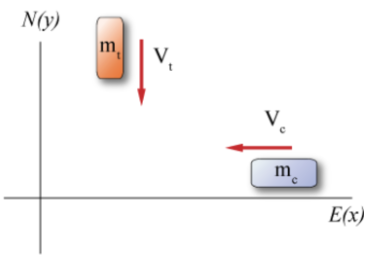# Problem:  A car with mass mc = 1134 kg is traveling west through an intersection at a magnitude of velocity of vc = 9.5 m/s when a truck of mass mt 1752 kg traveling south at vt = 8.8 m/s fails to yield and collides with the car. The vehicles become stuck together and slide on the asphalt, which has a coefficient of friction of μk = 0.5.A) Write an expression for the velocity of the system after the collision, in terms of the variables given in the problem statement and the unit vectors i and jB) How far, in meters, will the vehicles slide after the collision?

###### FREE Expert Solution

Law of conservation of momentum:

$\overline{)\begin{array}{rcl}{\mathbf{P}}_{\mathbf{0}}& {\mathbf{=}}& {\mathbf{P}}_{\mathbf{f}}\\ \begin{array}{rc}{\mathbf{m}}_{\mathbf{c}}{\mathbf{v}}_{\mathbf{c}}\mathbf{\left(}\mathbf{-}\stackrel{\mathbf{^}}{\mathbf{i}}& \mathbf{\right)}\mathbf{+}{\mathbf{m}}_{\mathbf{t}}{\mathbf{v}}_{\mathbf{t}}\mathbf{\left(}\mathbf{-}\stackrel{\mathbf{^}}{\mathbf{j}}\mathbf{\right)}\end{array}& {\mathbf{=}}& \mathbf{\left(}{\mathbf{m}}_{\mathbf{c}}\mathbf{+}{\mathbf{m}}_{\mathbf{t}}\mathbf{\right)}{\mathbf{v}}_{\mathbf{c}\mathbf{t}}\end{array}}$

We'll also use the kinematic equation;

$\overline{){{{\mathbf{v}}}_{{\mathbf{f}}}}^{{\mathbf{2}}}{\mathbf{=}}{{{\mathbf{v}}}_{{\mathbf{0}}}}^{{\mathbf{2}}}{\mathbf{+}}{\mathbf{2}}{\mathbf{a}}{\mathbf{∆}}{\mathbf{x}}}$###### Problem Details

A car with mass mc = 1134 kg is traveling west through an intersection at a magnitude of velocity of vc = 9.5 m/s when a truck of mass mt 1752 kg traveling south at vt = 8.8 m/s fails to yield and collides with the car. The vehicles become stuck together and slide on the asphalt, which has a coefficient of friction of μk = 0.5.A) Write an expression for the velocity of the system after the collision, in terms of the variables given in the problem statement and the unit vectors i and j

B) How far, in meters, will the vehicles slide after the collision?